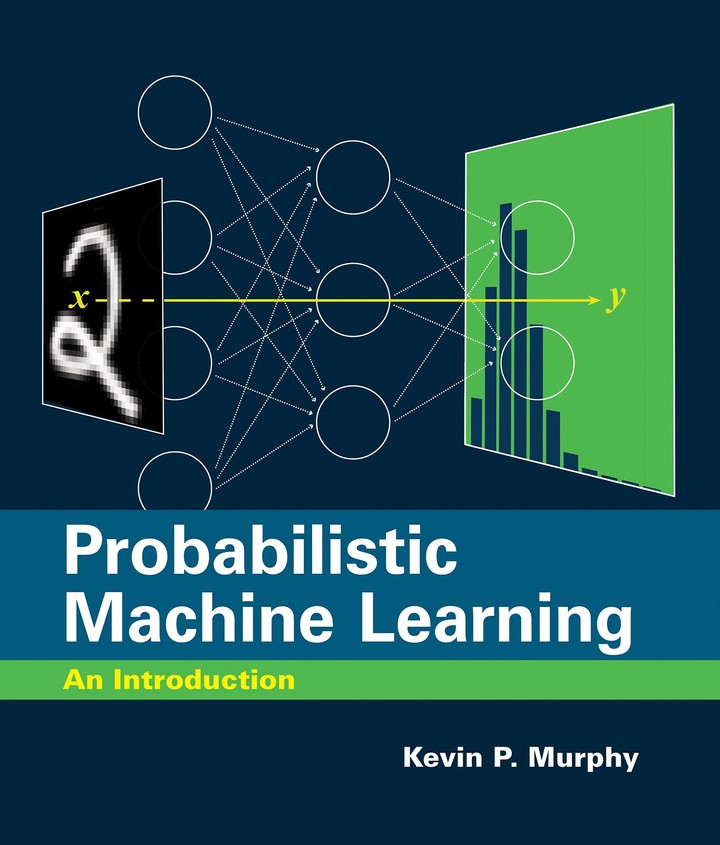# Course: Introduction to Machine Learning

(SoSe2023)Class timing: The academic quarter is applied here! This means the lecture will start 15 minutes after the said time.

### Course Description

Machine Learning has gained a lot of momentum within development organizations that are actively looking for innovative solutions to leverage their data to identify new levels of understanding their operations and processes. Machine learning is a subfield of Artificial Intelligence where the machine learns from data rather than from explicit programming. Machine Learning applications are likely to be part of your daily life without you being aware of it! Take reCAPTCHA as an example that you might face on a daily basis! This course will walk you through the basics of machine learning and explain how to formulate machine learning problems that are relevant to a wide range of applications. The class will be self-contained; a brief review on probability and information theory will be given before we dive into the main topics; linear and logistic regression, neural networks, non-parametric models, and unsupervised learning.

### Course Objectives

Successful students should

• gain a basic understanding of machine learning theory
• identify the strengths and weaknesses of various machine learning algorithms
• be able to formulate machine learning problems that are relevant to a wide range of applications
• be able to solve moderately complex problems using machine learning algorithms
• be able to apply, adapt and optimize machine learning algorithms to real-world problems
• be able to report various performance measures and evaluation metrics

### Prerequisites

• Linear Algebra
• Probability Theory
• Programming in Python

### Course Structure and grading policy (tentative):

• Assignments (10%): You will be occasionally given assigments or quizzes which contribute to 10% of your grade
• Lab. (20%): You will be given a few lab assignments to have hands-on experience with programming in python
• Midterm Exam (20%)
• Final Exam/Class Project (50%)

### Schedule

29.09.2022 Introduction (Ch1) Lecture01
06.10.2022 Foundations: Probability (Ch2) Lecture02 Statistics (Ch4) + Decicion Theory (Ch5)
13.10.2022 Foundations: Probability (Ch3) Assignment01 Information Theory (Ch6) + Linear Algebra (Ch7) + Optimization (Ch8)
20.10.2022 Linear Models: Logistic Regression (Ch10) Lecture03
27.10.2022 Linear Models: Logistic Regression (Ch10) – Cont. Assignment02 Linear Discriminative Analysis (Ch9)
03.11.2022 Linear Models: Linear Regression (Ch11) Lecture04 Generalized Linear Models (Ch12)
10.11.2022 Linear Models: Linear Regression (Ch11) – Cont. Lab01 Generalized Linear Models (Ch12)
17.11.2022 Mid-term Evaluation
01.12.2022 Neural Networks: NNs for Tabular Data (Ch13) Lecture05
08.12.2022 Neural Networks: NNs for Tabular Data (Ch13) – Cont. + Lab Lab02
15.12.2022 Neural Networks: NNs for Imaging Data (Ch14) Lecture06
22.12.2022 Beyond Supervised Learning: Dimensionality Reduction (Ch20) + Clustering (Ch21) Lecture07 Recommender Systems (Ch22)
29.12.2022 Hands-on Experience on the projects
05.01.2023 Course Recap. Lecture08
19.01.2023 Final Project Evaluation

### Contact the organizers

If you have any questions regarding the course, please do not hesitate to contact Prof. Dr. Shadi Albarqouni!